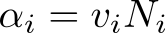Close

# Population Balance Modelling

#### Guide to CFD for Polydisperse Flows

1. Disperse Multiphase Flows
2. Polydisperse Multiphase Flows
3. Population Balance Modelling
4. Population Balance Modelling in OpenFOAM

This article provides the basic concepts behind the population balance model based on the method of classes, used for the simulation of polydisperse multiphase flows. A detailed theoretical description can be found in the associated publication by Lehnigk et al., AIChE J, 2022, Vol. 68(3), e17539.

## Number density function

The method solves a population balance equation, an “integro-partial differential equation” for the number density function, nv, for the distribution of particle volume, v. The number density function is the number of particles per unit volume with a property (here  v, in the case of  nv) within a range of values (here, v), normalised by the range. For example, if there are 24 air bubbles per m3 with bubble volumes in the range v = 3-5 mm3, the average number density corresponding to v = 4 mm3 (the midpoint) would be  nv = 24/((5-3) m3·mm3) = 12 particles/(m3·mm3).

The population balance equation treats nv as a continuous function corresponding to the limit of interval ranges → 0. It describes the evolution of nv due to effects of density and phase changes, coalescence and breakup. The method of classes is an approximate method to solving this equation. Together with suitable models for coalescence and breakup frequencies, the technique forms a population balance model.The method of classes takes its name from classification of entities into groups. Here, the number density function is split into M intervals, or size groups (i = 1, 2, … M), over ranges of particle volumes vi , with each group assigned a single representative particle volume vi . The number density function can then be integrated over any subinterval  vi to yield the number concentration Ni of particles in the corresponding size group i :The same integration is carried out for the entire population balance equation, which leads to a set of transport equations for the individual number concentrations. The arising source terms are written in a way that the conservation of the total volume and number of particles is ensured, irrespective of how the particle size domain is discretized.

## Size group fractions

Each number concentration is then converted into a volume concentration, αi , by multiplying it with the representative particle volume vi, by:The sum of volume concentrations yields the phase fraction α of the dispersed phase:The phase fraction α of the dispersed phase is thereby known from the number density function, so, in theory, there is no need to solve a transport equation for α . However, from a numerical standpoint it is critical that α is strictly bounded between 0 and 1 and that mass conservation is obeyed. Therefore in practice multiphaseEulerFoam solves a transport equation for α  which ensures conservation and, by using MULES (multidimensional universal limited explicit solver), boundedness is enforced.

With the phase fractions already solved for, the sole task of the population balance model is to determine how the dispersed phase elements are distributed with respect to size. This is achieved by transforming the transport equations for the number concentrations into equations for size group fractions fi which are bounded between zero and one and sum up to unity:## Sauter diameter

The interfacial models in the Euler-Euler model of multiphase flow describing the exchange of mass, momentum and energy rely on a surface area averaged diameter, also referred to as the Sauter diameter ds. From the size group fractions, d is calculated from the equivalent diameters di of each size group, e.g. di = (6vi /π)1/3 for spherical particles.The next article describes the basics of setting up a polydisperse multiphase flow simulation within multiphaseEulerFoam.